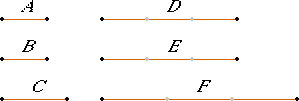# Proposition 7

Equal magnitudes have to the same the same ratio; and the same has to equal magnitudes the same ratio.

Let A and B be equal magnitudes and C an arbitrary magnitude.

I say that each of the magnitudes A and B has the same ratio to C, and C has the same ratio to each of the magnitudes A and B.Take equimultiples D and E of A and B, and take an arbitrary multiple F of C.

Then, since D is the same multiple of A that E is of B, and A equals B, therefore D equals E.

But F is another, arbitrary, magnitude. If therefore D is in excess of F, then E is also in excess of F; if equal, equal; and, if less, less.

V.Def.5

And D and E are equimultiples of A and B, while F is another, arbitrary, multiple of C, therefore A is to C as B is to C.

I say next that C also has the same ratio to each of the magnitudes A and B.

With the same construction, we can prove similarly that D equals E, and F is some other magnitude. If therefore F is in excess of D, it is also in excess of E; if equal, equal; and, if less, less.

V.Def.5

And F is a multiple of C, while D and E are other, arbitrary, equimultiples of A and B, therefore C is to A as C is to B.

Therefore, equal magnitudes have to the same the same ratio; and the same has to equal magnitudes the same ratio.

Q.E.D.

# Corollary

From this it is clear that, if any magnitudes are proportional, then they are also proportional inversely.

## Guide

This proposition says that if a = b, then a : c = b : c, and c : a = c : b. The proposition is evident. Its converse is given in V.9.

The corollary is misplaced. There is nothing relevant in the proposition. There’s no way it could yield the corollary since the proposition requires all the magnitudes to be of the same kind and the corollary doesn’t. But the corollary is valid, and it follows easily from definition V.Def.5.

#### Use of this proposition

Such a basic property of ratios as this is used frequently when ratios are mentioned. It is used in a few times in Book V starting with V.10, frequently in Book VI, and occasionally in later books.